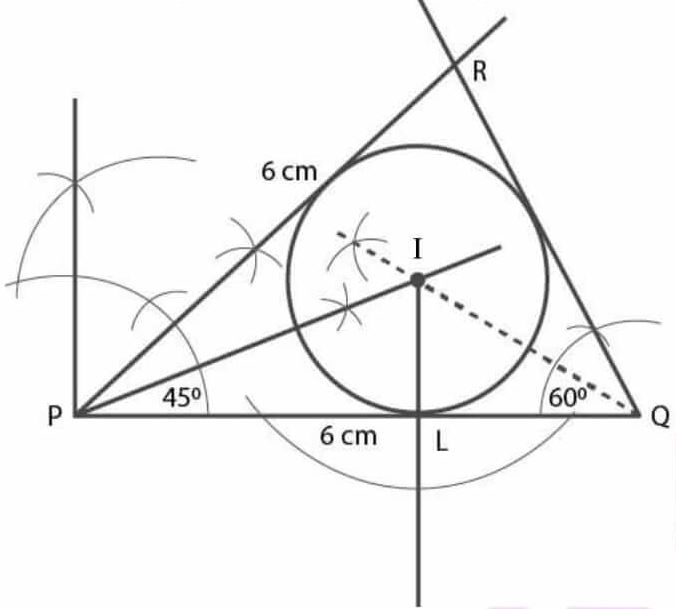# Selina Solutions Concise Maths Class 7 Chapter 15: Triangles Exercise 15C

Selina Solutions Concise Maths Class 7 Chapter 15 Triangles Exercise 15C has stepwise answers, which help in the construction of triangles. The solutions contain explanations in simple language, based on the understanding abilities of students. Important formulas, shortcut tricks and steps are covered in the PDF to boost the exam preparation of students. The solutions created are 100% accurate, as per the ICSE exam pattern. In order to improve the academic performance, Selina Solutions Concise Maths Class 7 Chapter 15 Triangles Exercise 15C, PDF can be downloaded from the links which are provided here.

## Selina Solutions Concise Maths Class 7 Chapter 15: Triangles Exercise 15C Download PDF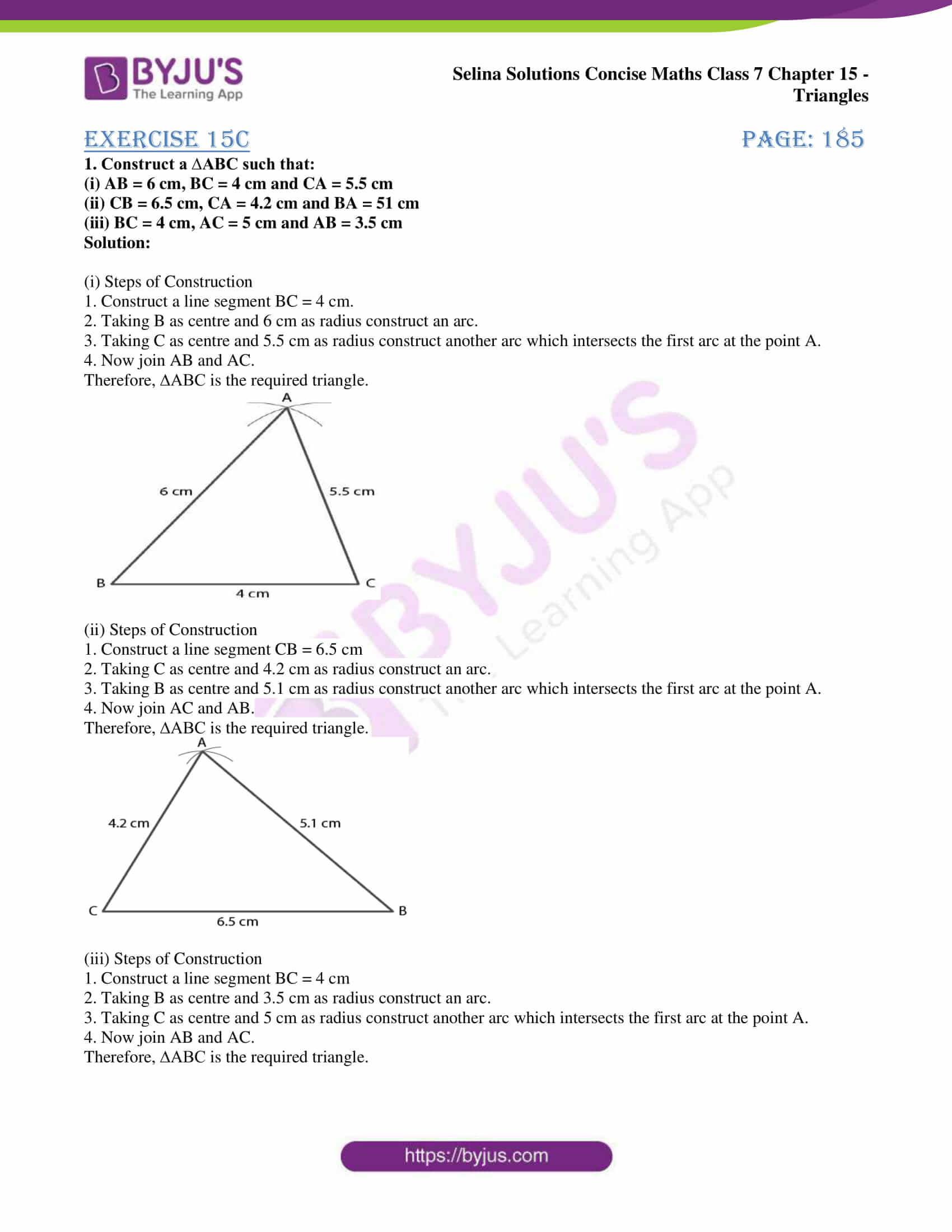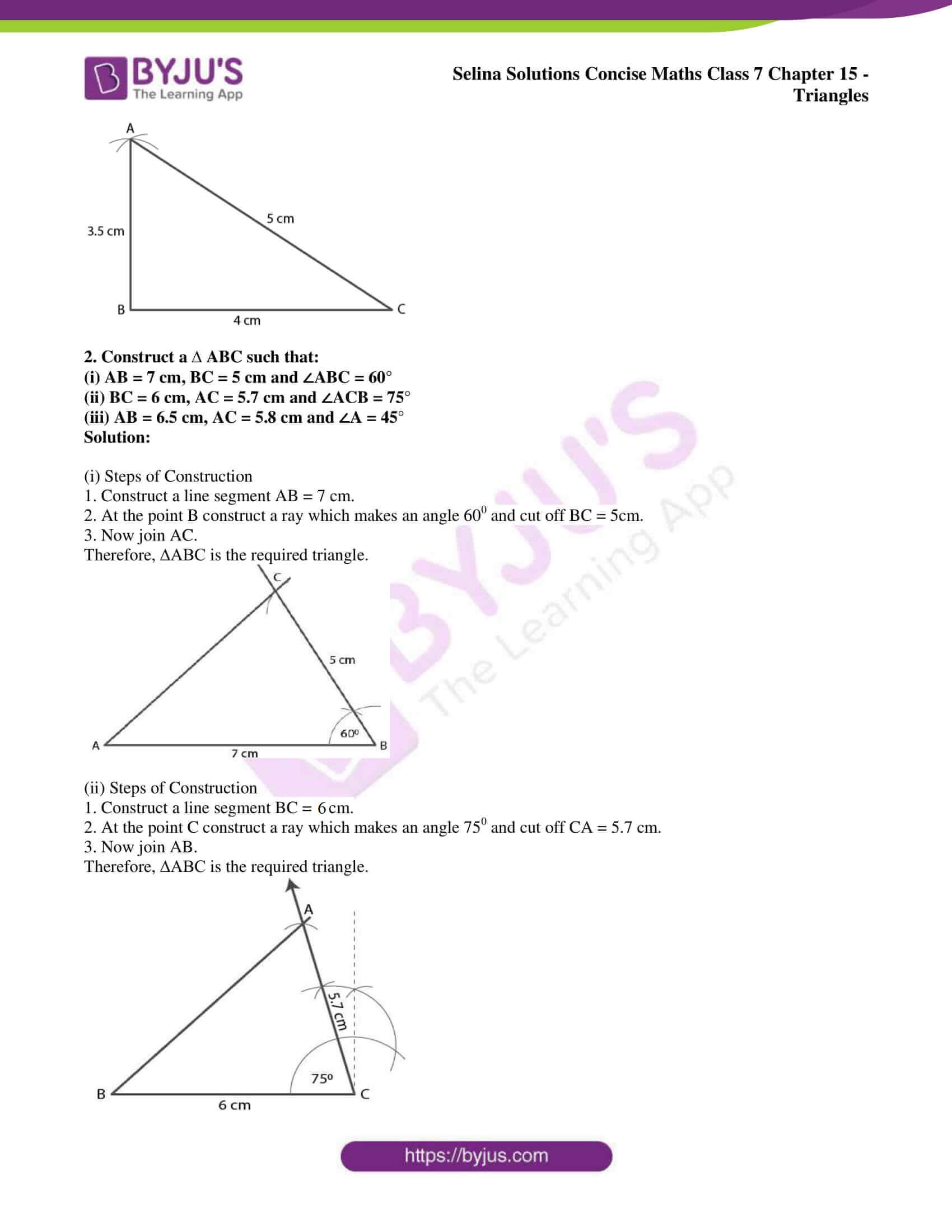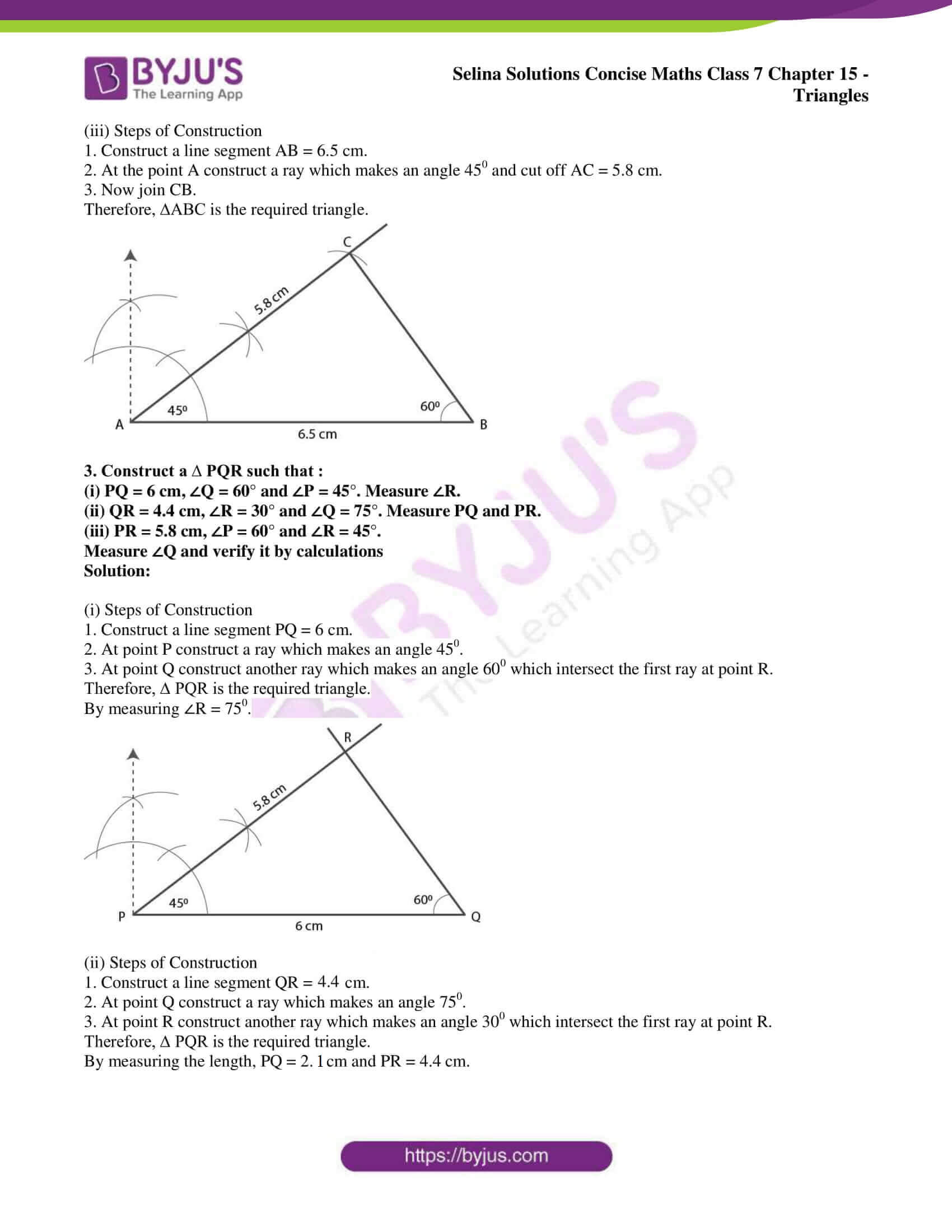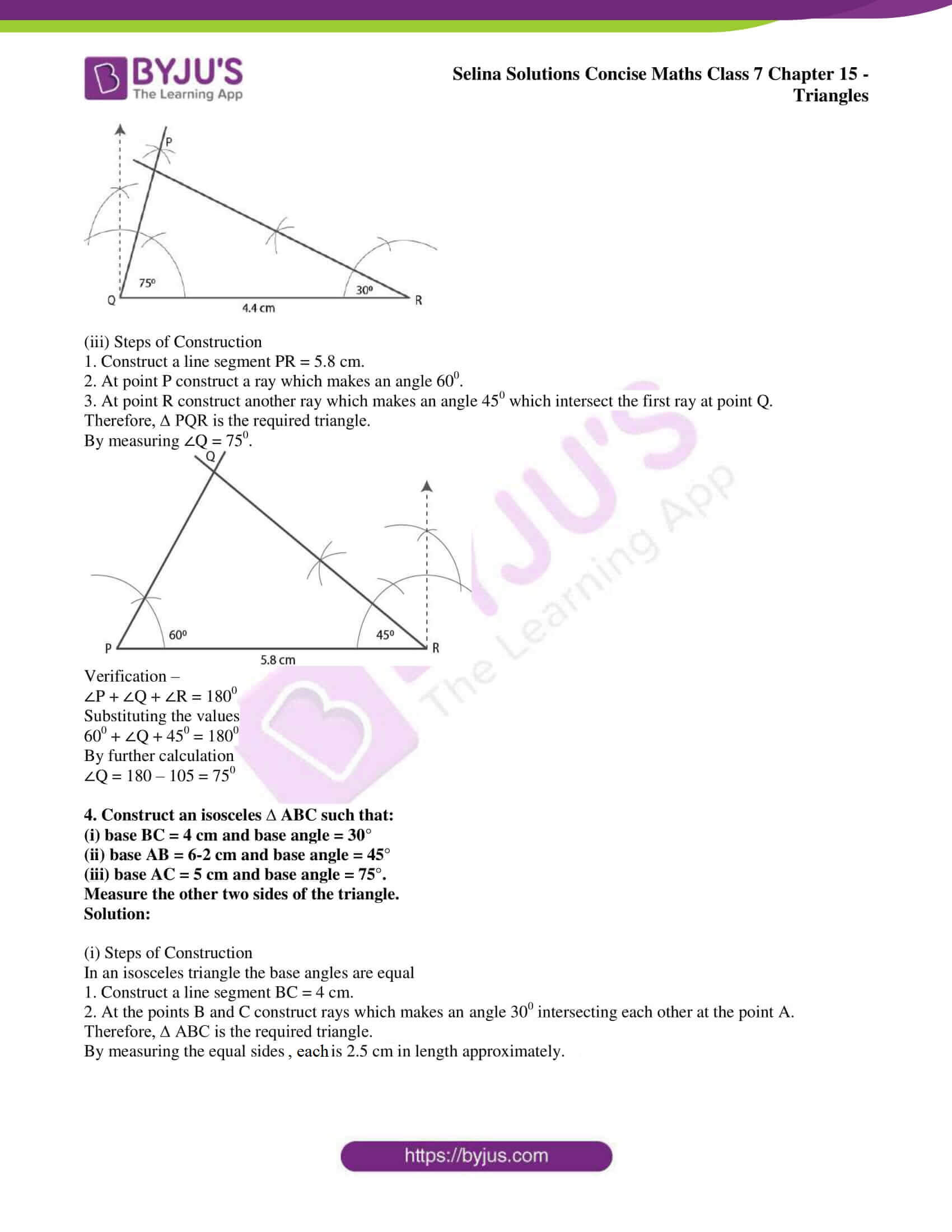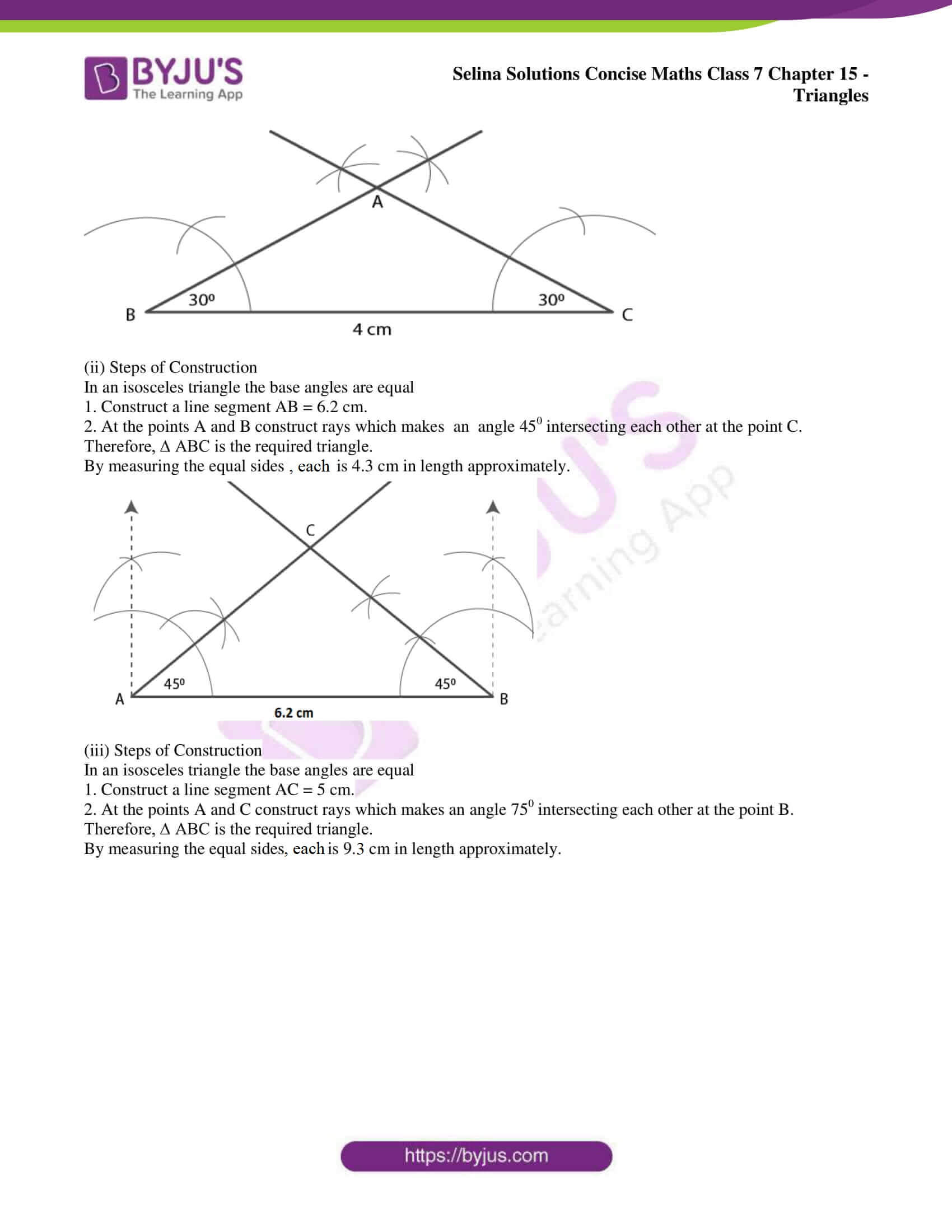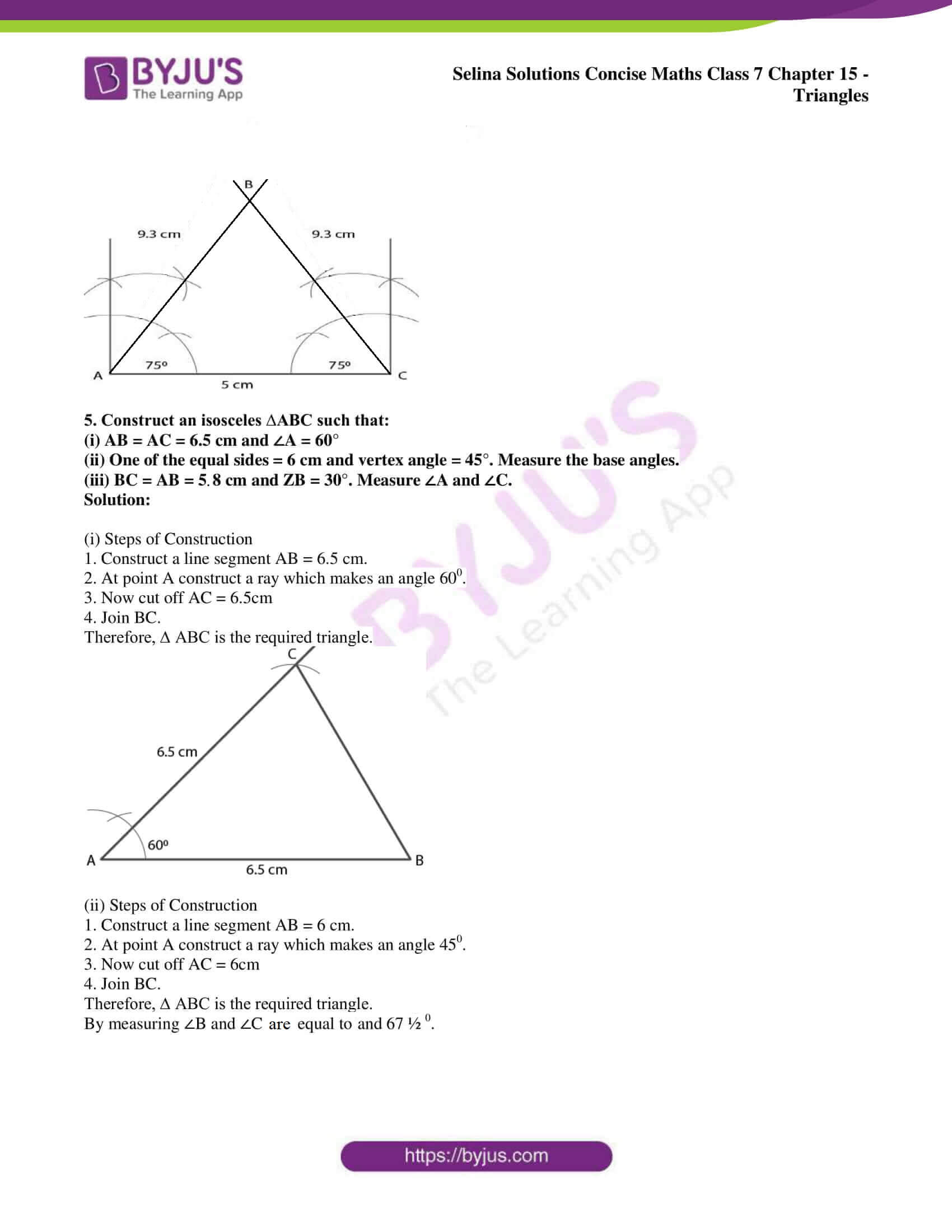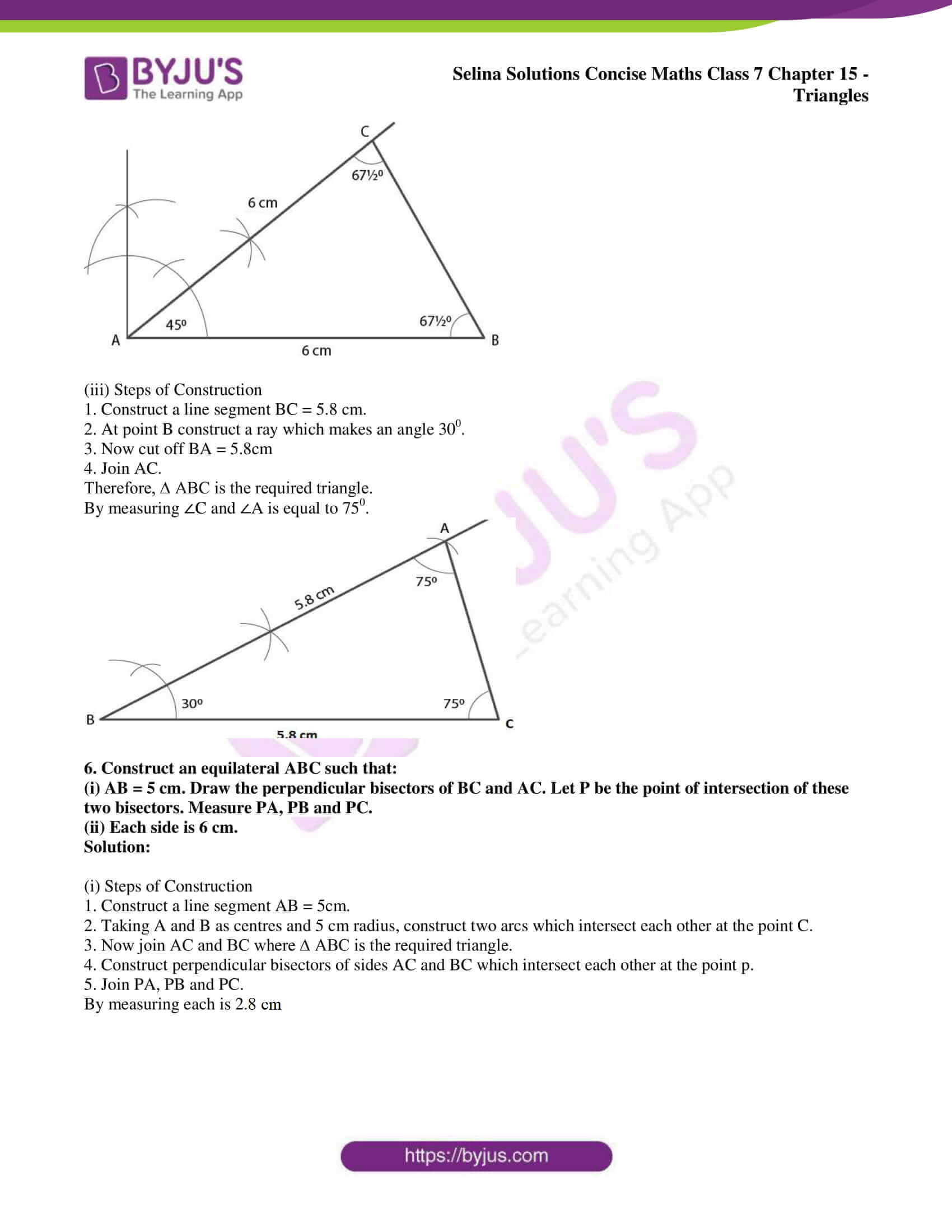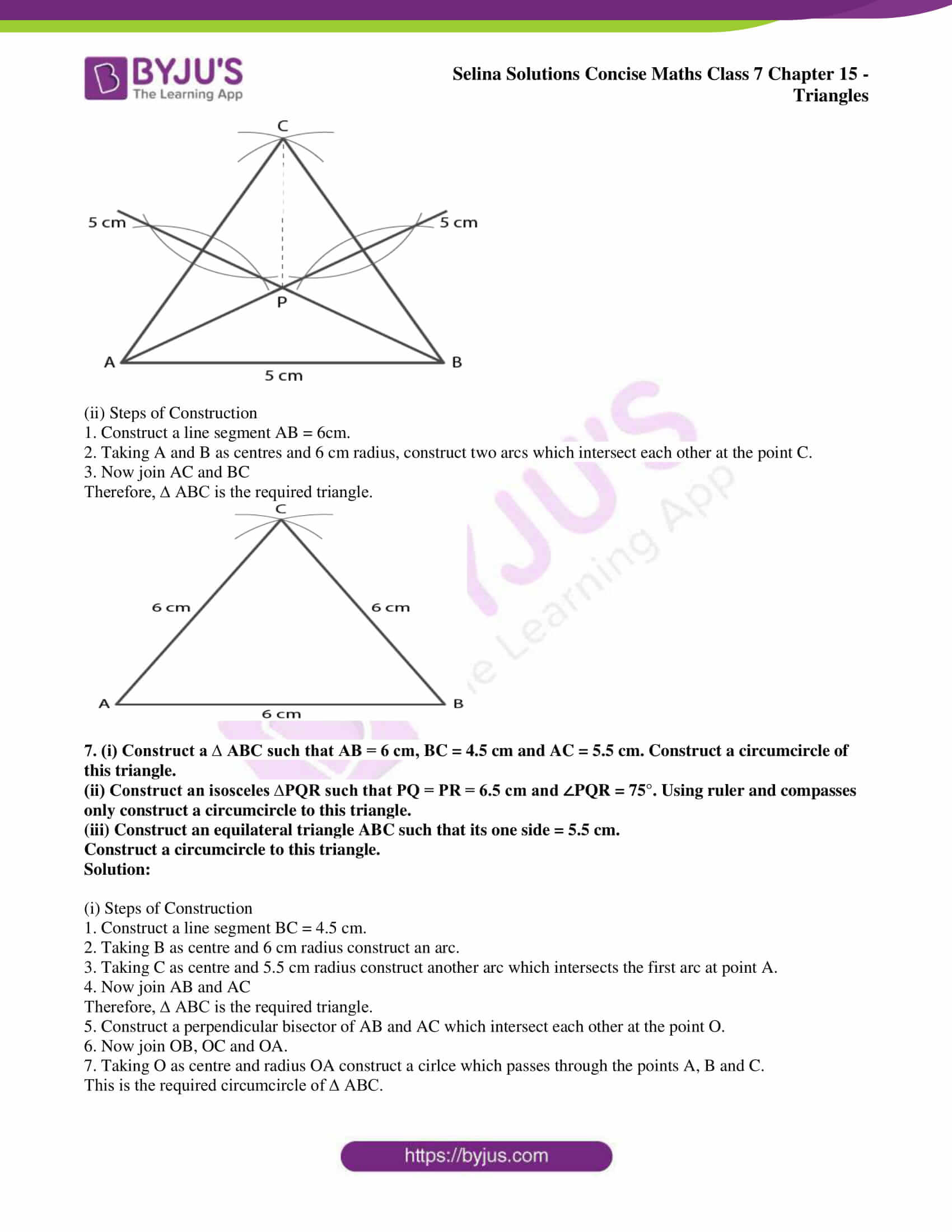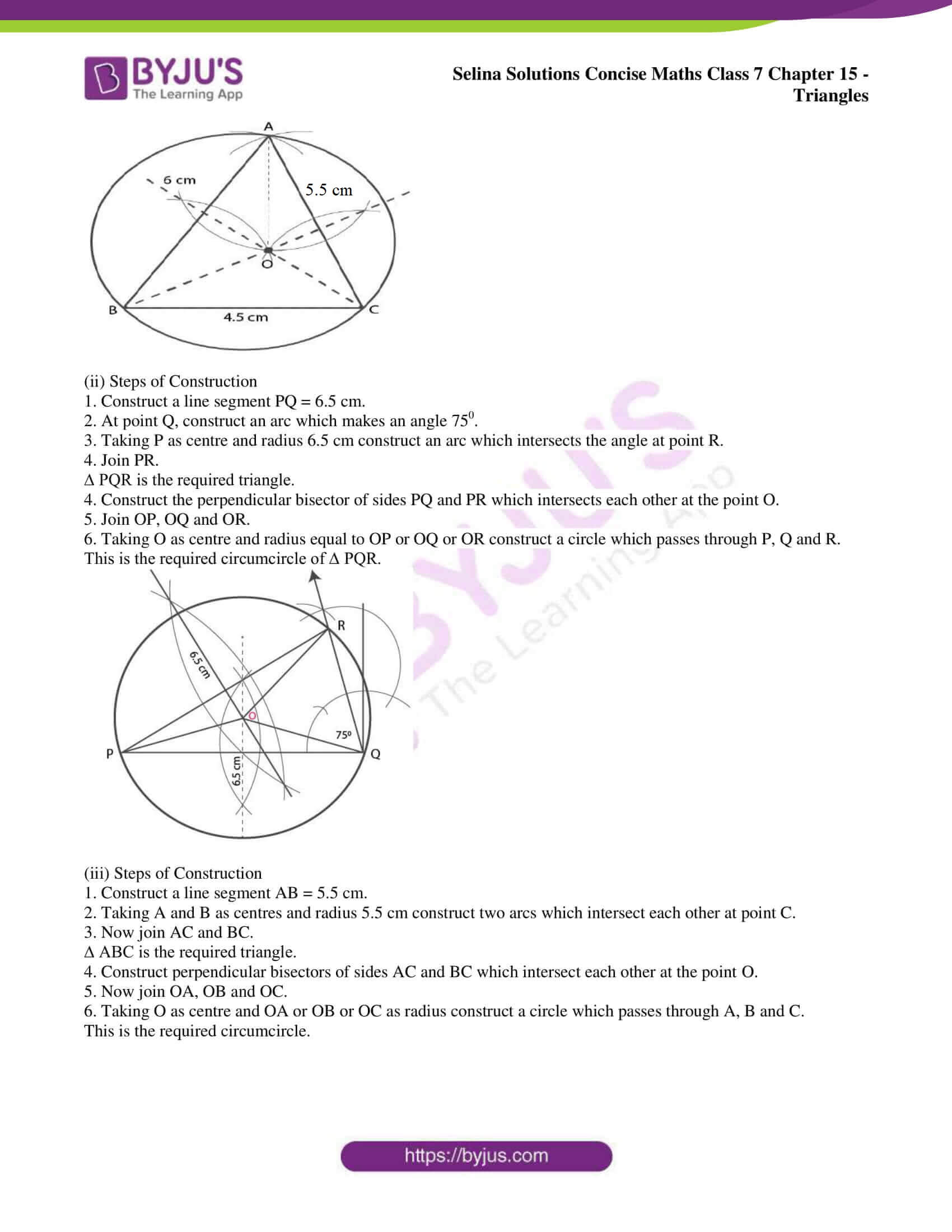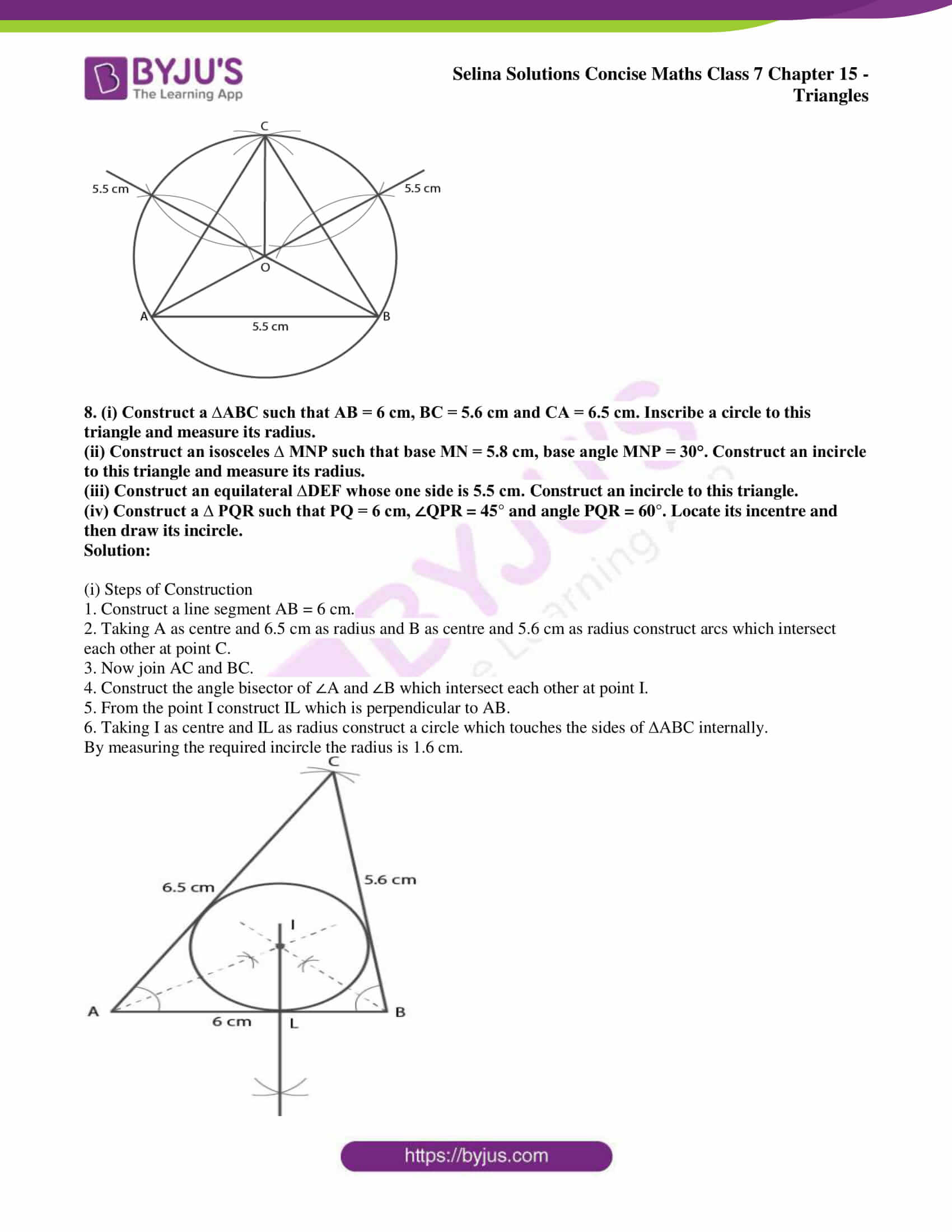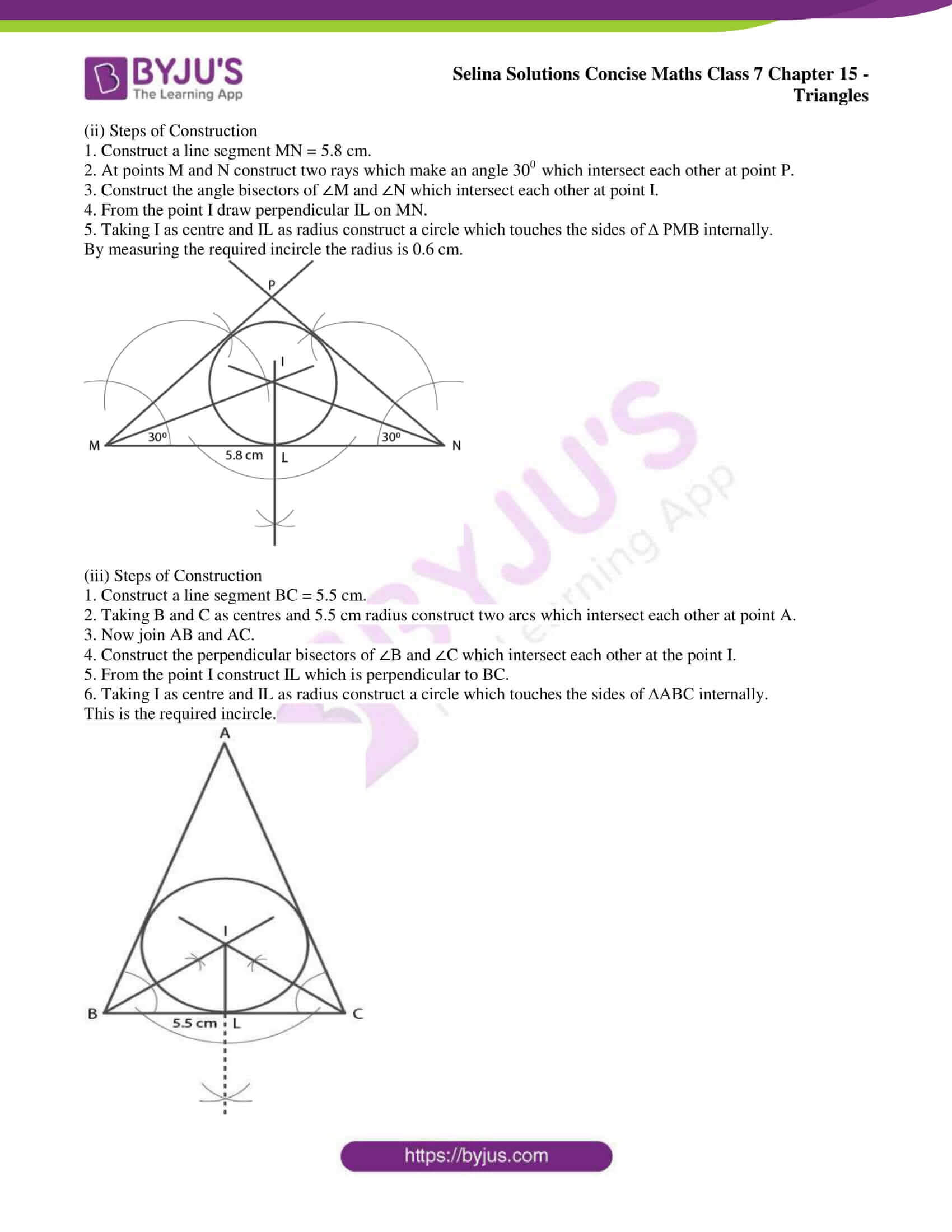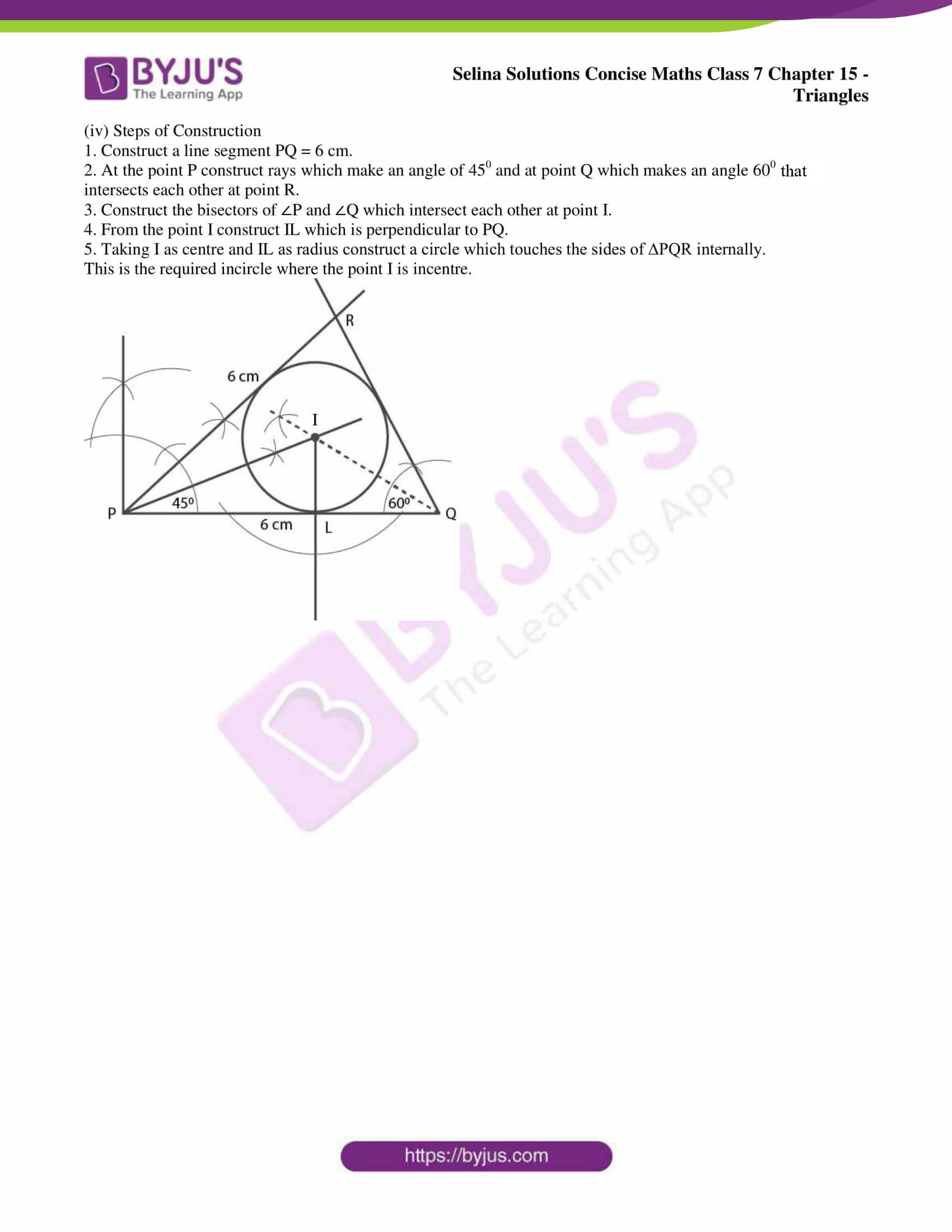### Access other exercises of Selina Solutions Concise Maths Class 7 Chapter 15: Triangles

Exercise 15A Solutions

Exercise 15B Solutions

### Access Selina Solutions Concise Maths Class 7 Chapter 15: Triangles Exercise 15C

#### Exercise 15C page: 185

1. Construct a ∆ABC such that:
(i) AB = 6 cm, BC = 4 cm and CA = 5.5 cm
(ii) CB = 6.5 cm, CA = 4.2 cm and BA = 51 cm
(iii) BC = 4 cm, AC = 5 cm and AB = 3.5 cm

Solution:

(i) Steps of Construction

1. Construct a line segment BC = 4 cm.

2. Taking B as centre and 6 cm as radius construct an arc.

3. Taking C as centre and 5.5 cm as radius construct another arc which intersects the first arc at the point A.

4. Now join AB and AC.

Therefore, ∆ABC is the required triangle.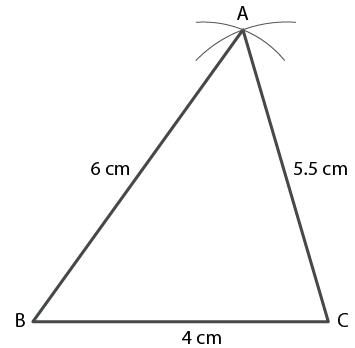(ii) Steps of Construction

1. Construct a line segment CB = 6.5 cm

2. Taking C as centre and 4.2 cm as radius construct an arc.

3. Taking B as centre and 5.1 cm as radius construct another arc which intersects the first arc at the point A.

4. Now join AC and AB.

Therefore, ∆ABC is the required triangle.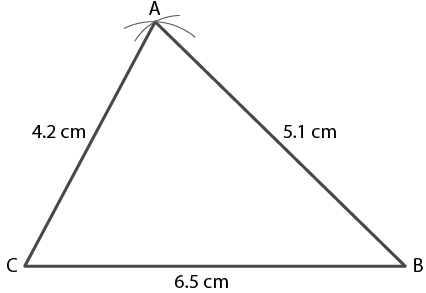(iii) Steps of Construction

1. Construct a line segment BC = 4 cm

2. Taking B as centre and 3.5 cm as radius construct an arc.

3. Taking C as centre and 5 cm as radius construct another arc which intersects the first arc at the point A.

4. Now join AB and AC.

Therefore, ∆ABC is the required triangle.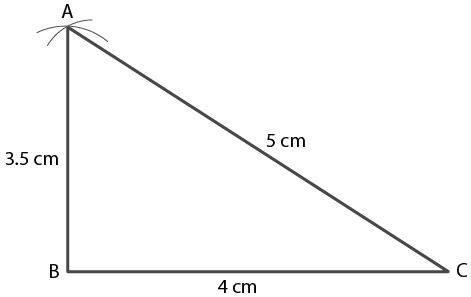2. Construct a ABC such that:
(i) AB = 7 cm, BC = 5 cm and ∠ABC = 60°
(ii) BC = 6 cm, AC = 5.7 cm and ∠ACB = 75°
(iii) AB = 6.5 cm, AC = 5.8 cm and ∠A = 45°

Solution:

(i) Steps of Construction

1. Construct a line segment AB = 7 cm.

2. At the point B construct a ray which makes an angle 600 and cut off BC = 5cm.

3. Now join AC.

Therefore, ∆ABC is the required triangle.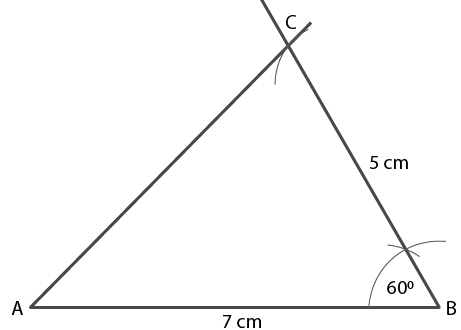(ii) Steps of Construction

1. Construct a line segment BC = 6 cm.

2. At the point C construct a ray which makes an angle 750 and cut off CA = 5.7 cm.

3. Now join AB.

Therefore, ∆ABC is the required triangle.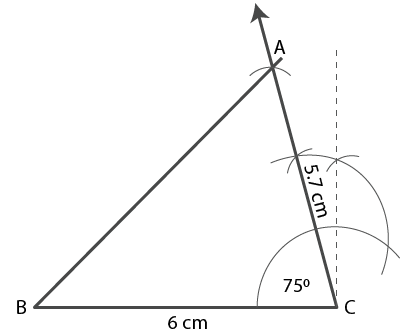(iii) Steps of Construction

1. Construct a line segment AB = 6.5 cm.

2. At the point A construct a ray which makes an angle 450 and cut off AC = 5.8 cm.

3. Now join CB.

Therefore, ∆ABC is the required triangle.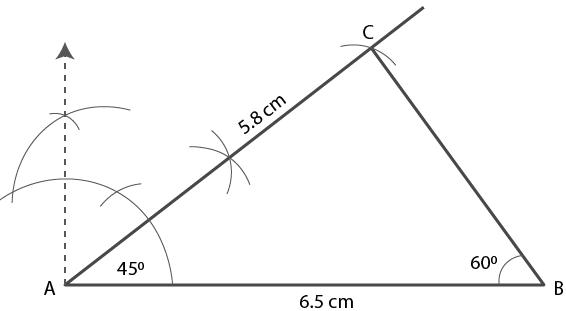3. Construct a ∆ PQR such that :
(i) PQ = 6 cm, ∠Q = 60° and ∠P = 45°. Measure ∠R.
(ii) QR = 4.4 cm, ∠R = 30° and ∠Q = 75°. Measure PQ and PR.
(iii) PR = 5.8 cm, ∠P = 60° and ∠R = 45°.
Measure ∠Q and verify it by calculations

Solution:

(i) Steps of Construction

1. Construct a line segment PQ = 6 cm.

2. At point P construct a ray which makes an angle 450.

3. At point Q construct another ray which makes an angle 600 which intersect the first ray at point R.

Therefore, ∆ PQR is the required triangle.

By measuring ∠R = 750.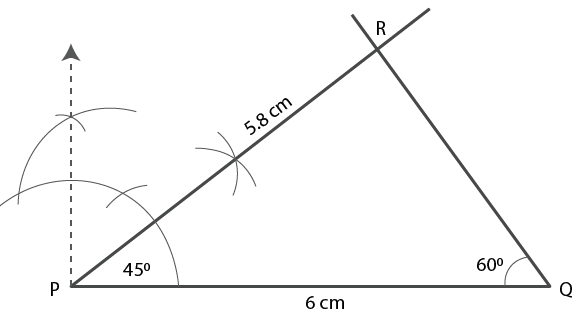(ii) Steps of Construction

1. Construct a line segment QR = 4.4 cm.

2. At point Q construct a ray which makes an angle 750.

3. At point R construct another ray which makes an angle 300 which intersect the first ray at point R.

Therefore, ∆ PQR is the required triangle.

By measuring the length, PQ = 2.1 cm and PR = 4.4 cm.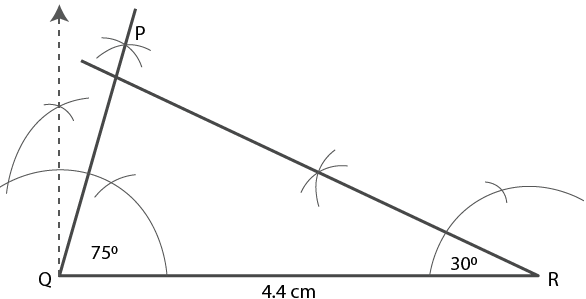(iii) Steps of Construction

1. Construct a line segment PR = 5.8 cm.

2. At point P construct a ray which makes an angle 600.

3. At point R construct another ray which makes an angle 450 which intersect the first ray at point Q.

Therefore, ∆ PQR is the required triangle.

By measuring ∠Q = 750.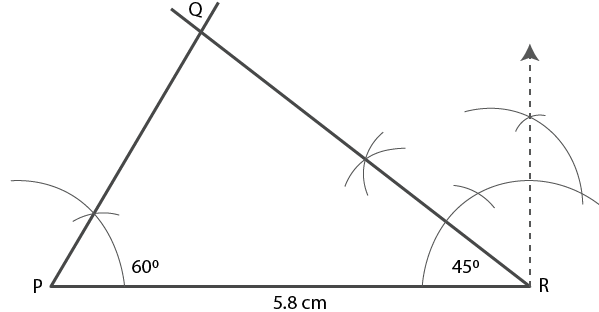Verification –

∠P + ∠Q + ∠R = 1800

Substituting the values

600 + ∠Q + 450 = 1800

By further calculation

∠Q = 180 – 105 = 750

4. Construct an isosceles ABC such that:
(i) base BC = 4 cm and base angle = 30°
(ii) base AB = 6.2 cm and base angle = 45°
(iii) base AC = 5 cm and base angle = 75°.
Measure the other two sides of the triangle.

Solution:

(i) Steps of Construction

In an isosceles triangle the base angles are equal

1. Construct a line segment BC = 4 cm.

2. At the points B and C construct rays which makes an angle 300 intersecting each other at the point A.

Therefore, ∆ ABC is the required triangle.

By measuring the equal sides, each is 2.5 cm in length approximately.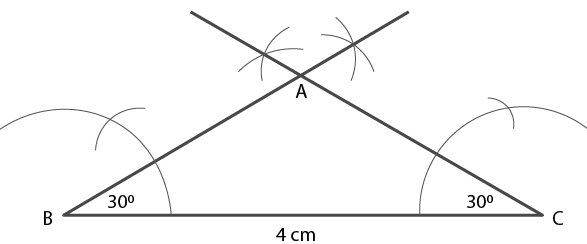(ii) Steps of Construction

In an isosceles triangle the base angles are equal

1. Construct a line segment AB = 6.2 cm.

2. At the points A and B construct rays which makes an angle 450 intersecting each other at the point C.

Therefore, ∆ ABC is the required triangle.

By measuring the equal sides, each is 4.3 cm in length approximately.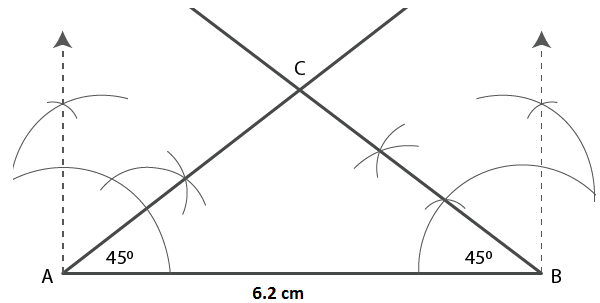(iii) Steps of Construction

In an isosceles triangle the base angles are equal

1. Construct a line segment AC = 5 cm.

2. At the points A and C construct rays which makes an angle 750 intersecting each other at the point B.

Therefore, ∆ ABC is the required triangle.

By measuring the equal sides, each is 9.3 cm in length approximately.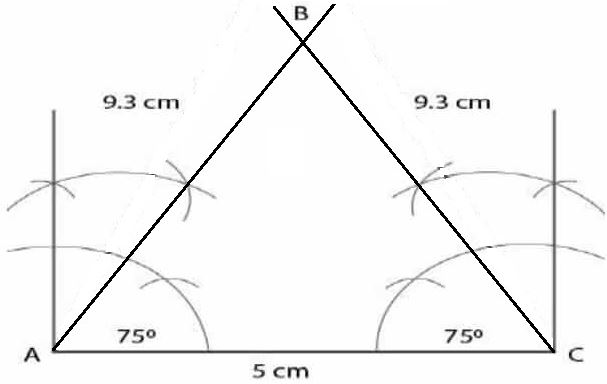5. Construct an isosceles ∆ABC such that:
(i) AB = AC = 6.5 cm and ∠A = 60°
(ii) One of the equal sides = 6 cm and vertex angle = 45°. Measure the base angles.
(iii) BC = AB = 5-8 cm and ZB = 30°. Measure ∠A and ∠C.

Solution:

(i) Steps of Construction

1. Construct a line segment AB = 6.5 cm.

2. At point A construct a ray which makes an angle 600.

3. Now cut off AC = 6.5cm

4. Join BC.

Therefore, ∆ ABC is the required triangle.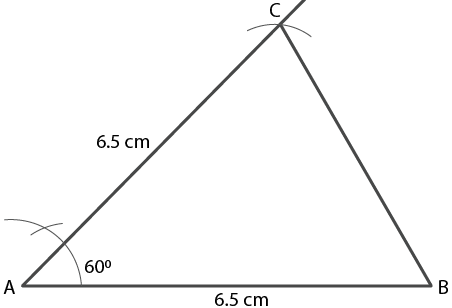(ii) Steps of Construction

1. Construct a line segment AB = 6 cm.

2. At point A construct a ray which makes an angle 450.

3. Now cut off AC = 6cm

4. Join BC.

Therefore, ∆ ABC is the required triangle.

By measuring ∠B and ∠C, both are equal to 67 ½ 0.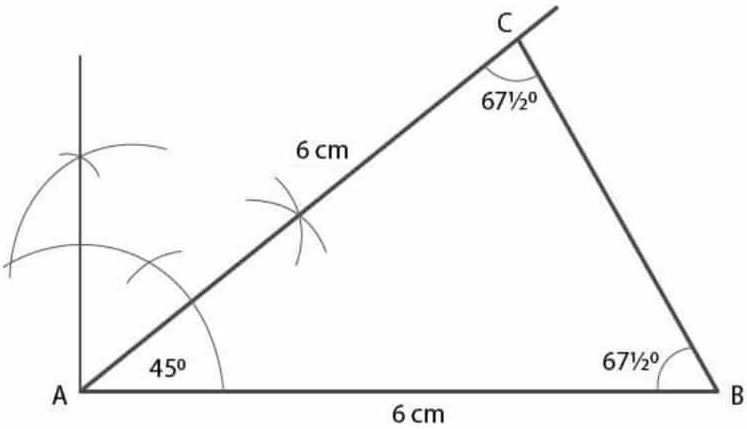(iii) Steps of Construction

1. Construct a line segment BC = 5.8 cm.

2. At point B construct a ray which makes an angle 300.

3. Now cut off BA = 5.8cm

4. Join AC.

Therefore, ∆ ABC is the required triangle.

By measuring ∠C and ∠A is equal to 750.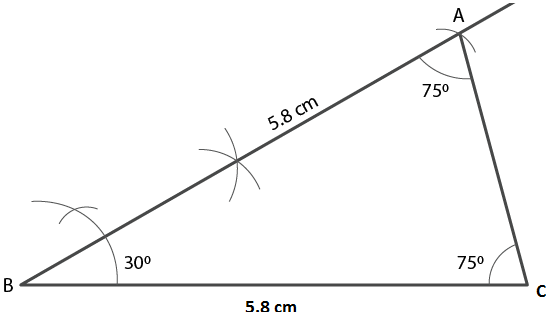6. Construct an equilateral triangle ABC such that:
(i) AB = 5 cm. Draw the perpendicular bisectors of BC and AC. Let P be the point of intersection of these two bisectors. Measure PA, PB and PC.
(ii) Each side is 6 cm.

Solution:

(i) Steps of Construction

1. Construct a line segment AB = 5cm.

2. Taking A and B as centres and 5 cm radius, construct two arcs which intersect each other at the point C.

3. Now join AC and BC where ∆ ABC is the required triangle.

4. Construct perpendicular bisectors of sides AC and BC which intersect each other at the point p.

5. Join PA, PB and PC.

By measuring each is 2.8 cm.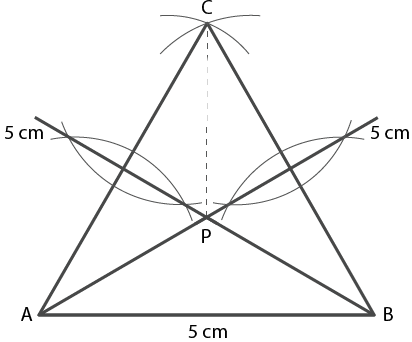(ii) Steps of Construction

1. Construct a line segment AB = 6cm.

2. Taking A and B as centres and 6 cm radius, construct two arcs which intersect each other at the point C.

3. Now join AC and BC

Therefore, ∆ ABC is the required triangle.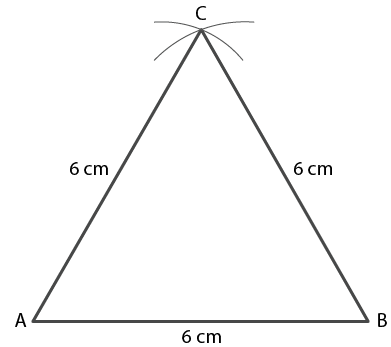7. (i) Construct a ∆ ABC such that AB = 6 cm, BC = 4.5 cm and AC = 5.5 cm. Construct a circumcircle of this triangle.
(ii) Construct an isosceles ∆PQR such that PQ = PR = 6.5 cm and ∠PQR = 75°. Using ruler and compasses only construct a circumcircle to this triangle.
(iii) Construct an equilateral triangle ABC such that its one side = 5.5 cm.
Construct a circumcircle to this triangle.

Solution:

(i) Steps of Construction

1. Construct a line segment BC = 4.5 cm.

2. Taking B as centre and 6 cm radius construct an arc.

3. Taking C as centre and 5.5 cm radius construct another arc which intersects the first arc at point A.

4. Now join AB and AC

Therefore, ∆ ABC is the required triangle.

5. Construct a perpendicular bisector of AB and AC which intersect each other at the point O.

6. Now join OB, OC and OA.

7. Taking O as centre and radius OA construct a cirlce which passes through the points A, B and C.

This is the required circumcircle of ∆ ABC.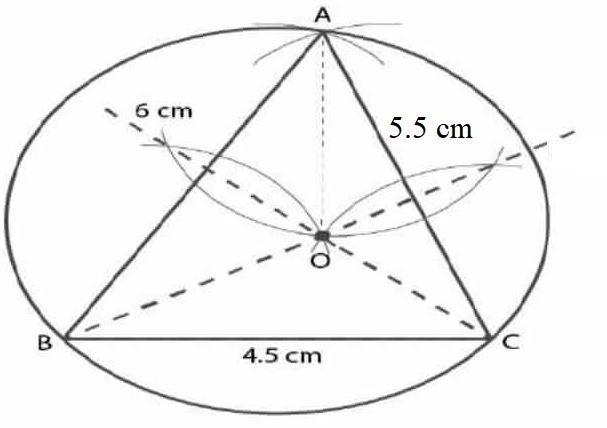(ii) Steps of Construction

1. Construct a line segment PQ = 6.5 cm.

2. At point Q, construct an arc which makes an angle 750.

3. Taking P as centre and radius 6.5 cm construct an arc which intersects the angle at point R.

4. Join PR.

∆ PQR is the required triangle.

4. Construct the perpendicular bisector of sides PQ and PR which intersects each other at the point O.

5. Join OP, OQ and OR.

6. Taking O as centre and radius equal to OP or OQ or OR construct a circle which passes through P, Q and R.

This is the required circumcircle of ∆ PQR.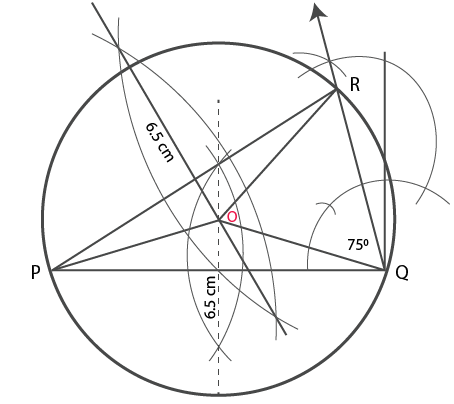(iii) Steps of Construction

1. Construct a line segment AB = 5.5 cm.

2. Taking A and B as centres and radius 5.5 cm construct two arcs which intersect each other at point C.

3. Now join AC and BC.

∆ ABC is the required triangle.

4. Construct perpendicular bisectors of sides AC and BC which intersect each other at the point O.

5. Now join OA, OB and OC.

6. Taking O as centre and OA or OB or OC as radius construct a circle which passes through A, B and C.

This is the required circumcircle.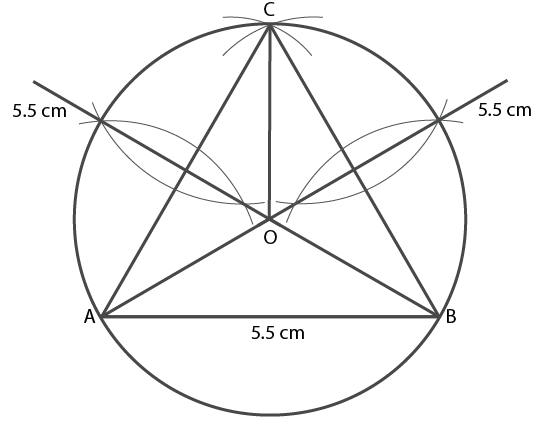8. (i) Construct a ∆ABC such that AB = 6 cm, BC = 5.6 cm and CA = 6.5 cm. Inscribe a circle to this triangle and measure its radius.
(ii) Construct an isosceles ∆ MNP such that base MN = 5.8 cm, base angle MNP = 30°. Construct an incircle to this triangle and measure its radius.
(iii) Construct an equilateral ∆DEF whose one side is 5.5 cm. Construct an incircle to this triangle.
(iv) Construct a ∆ PQR such that PQ = 6 cm, ∠QPR = 45° and angle PQR = 60°. Locate its incentre and then draw its incircle.

Solution:

(i) Steps of Construction

1. Construct a line segment AB = 6 cm.

2. Taking A as centre and 6.5 cm as radius and B as centre and 5.6 cm as radius construct arcs which intersect each other at point C.

3. Now join AC and BC.

4. Construct the angle bisector of ∠A and ∠B which intersect each other at point I.

5. From the point I construct IL which is perpendicular to AB.

6. Taking I as centre and IL as radius construct a circle which touches the sides of ∆ABC internally.

By measuring the required incircle the radius is 1.6 cm.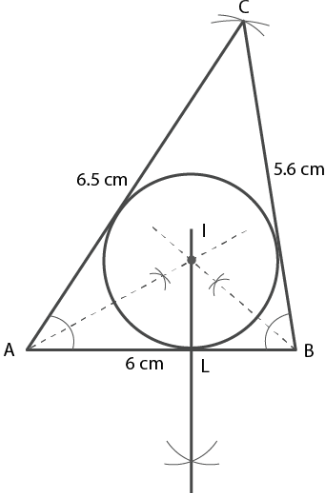(ii) Steps of Construction

1. Construct a line segment MN = 5.8 cm.

2. At points M and N construct two rays which make an angle 300 each intersecting each other at point P.

3. Construct the angle bisectors of ∠M and ∠N which intersect each other at point I.

4. From the point I draw perpendicular IL on MN.

5. Taking I as centre and IL as radius construct a circle which touches the sides of ∆ PMB internally.

By measuring the required incircle the radius is 0.6 cm.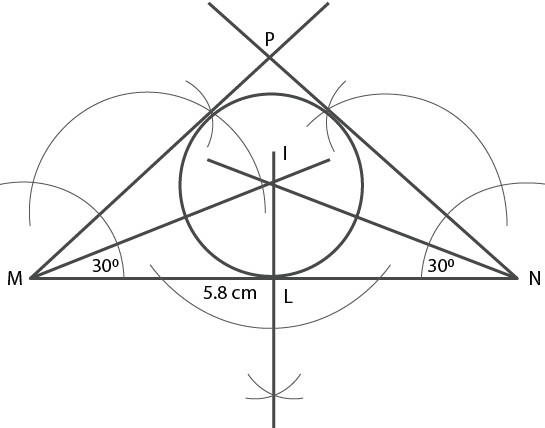(iii) Steps of Construction

1. Construct a line segment BC = 5.5 cm.

2. Taking B and C as centres and 5.5 cm radius construct two arcs which intersect each other at point A.

3. Now join AB and AC.

4. Construct the perpendicular bisectors of ∠B and ∠C which intersect each other at the point I.

5. From the point I construct IL which is perpendicular to BC.

6. Taking I as centre and IL as radius construct a circle which touches the sides of ∆ABC internally.

This is the required incircle.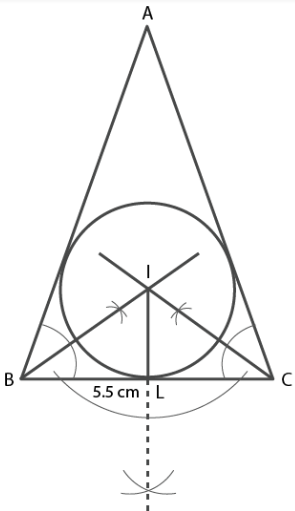(iv) Steps of Construction

1. Construct a line segment PQ = 6 cm.

2. At the point P construct rays which make an angle of 450 and at point Q which makes an angle 600 thats intersects each other at point R.

3. Construct the bisectors of ∠P and ∠Q which intersect each other at point I.

4. From the point I construct IL which is perpendicular to PQ.

5. Taking I as centre and IL as radius construct a circle which touches the sides of ∆PQR internally.

This is the required incircle where the point I is incentre.